# Logarithmic equations

## Summary and examples

Roughly speaking, logarithmic equations are equations containing a logarithmic function with the unknown variable as argument, see also Logarithmic functions and graphs.

An example of such an equation is: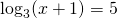The unknown variableis part of the argument of the logarithmic function, which has base 3. Before we start solving the equation we could first determine the domain of the equation. In this equation the domain is:Then solving the equation is straightforward. Using all kinds of rules and tricks we try to get an equation of the form: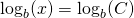From this equation we can deduce the solution: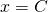The following four rules are very useful when we try to solve logarithmic equations:

1.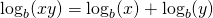2.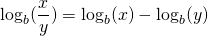3.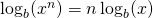4.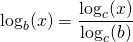Of course, for the base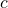the same conditions hold as for any base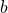.

Also the following rule is heavily used: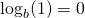Finally, it is always helpful to keep the definition of a logarithmic function in mind: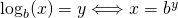##### Example 1

Solve: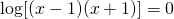The logarithmic function is defined if: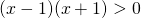thus if: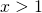or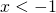This is called the domain of the function.

We can rewrite the equation as follows: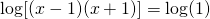and thus: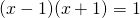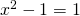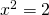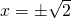Comparing these solutions with the earlier calculated domain, we find that both solutions satisfy the equation.

##### Example 2

Solve:The domain of the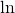-function is: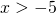We write for the equation: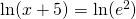resulting in: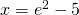This solution satisfies the equation.

##### Example 3

Solve: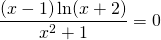The domain of the function in the left-hand side is (because of the-function):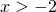If a fraction equals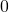, then the numerator is equal tois. So: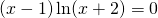It follows: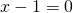or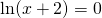and thus: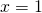orThe last equation can be rewritten as: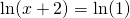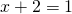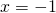The solutions are:orand these have to be combined with the domain:We may conclude that both solutions satisfy the equation.

##### Example 4

Solve: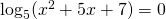First we determine the domain of the-functie. The argument has to be greater than: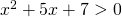The quadratic function in the left-hand side has an 'opens up' parabola as a graph and the determinant satisfies: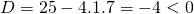meaning that the parabola has no intersection points with the-axis and the inequality above holds for all values of.

We can write the equation as: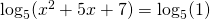and thus: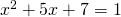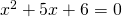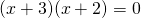This equation has the solutions: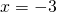or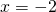The domain contains both values ofand thus both solutions satisfy the equation.

##### Example 5

Solve: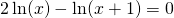The first logarithm is defined for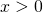, the second one for. Thus the equation is defined if both conditions are satisfied and thus the domain of the function in the left-hand side is.

We can rewrite the equation (using rule 3):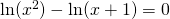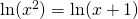It follows: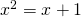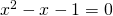We calculate the solutions of the quadratic equation using the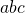-formula: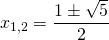Combining these values with the domain (), we may conclude that only the solution: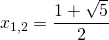satisfies the equation.

0